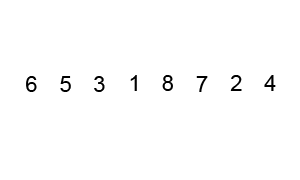# Quick Sort in Java using Divide and Conquer

In this program, you'll learn to implement Quick sort in Java. Quicksort is a divide and conquer algorithm. Quicksort first divides a large list into two smaller sub-lists: the low elements and the high elements. Quicksort can then recursively sort the sub-lists.

The key process in quickSort is partition(). Target of partitions is, given an array and an element x of array as pivot, put x at its correct position in sorted array and put all smaller elements (smaller than x) before x, and put all greater elements (greater than x) after x. All this should be done in linear time.

#### The steps are:

1. Pick an element, called a pivot, from the list.
2. Reorder the list so that all elements with values less than the pivot come before the pivot, while all elements with values greater than the pivot come after it (equal values can go either way). After this partitioning, the pivot is in its final position. This is called the partition operation.
3. Recursively sort the sub-list of lesser elements and the sub-list of greater elements.
The base case of the recursion are lists of size zero or one, which never need to be sorted.Wikipedia

#### When you run the program, the output will be:

`Before Sort : `
`32 54 29 51 48 69 47 58 89 71 `
`After Sort : `
`29 32 47 48 51 54 58 69 71 89 `